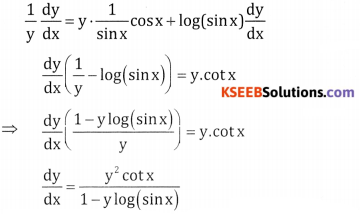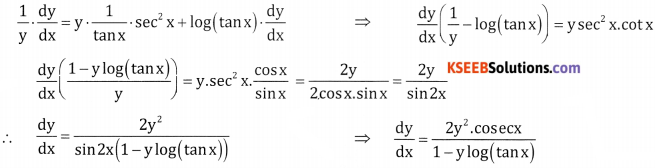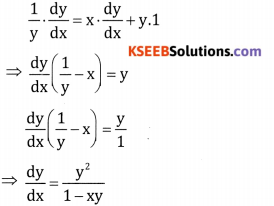# 2nd PUC Basic Maths Question Bank Chapter 18 Differential Calculus Ex 18.4

Students can Download Basic Maths Exercise 18.4 Questions and Answers, Notes Pdf, 2nd PUC Basic Maths Question Bank with Answers helps you to revise the complete Karnataka State Board Syllabus and score more marks in your examinations.

## Karnataka 2nd PUC Basic Maths Question Bank Chapter 18 Differential Calculus Ex 18.4

Part – A

2nd PUC Basic Maths Differential Calculus Ex 18.4 Three Marks Questions and Answers

Question 1.
If y = $$y=\sqrt{x+\sqrt{x+\sqrt{x \ldots \ldots \infty}}}$$ then prove that $$\frac{d y}{d x}=\frac{1}{2 y-1}$$
Given $$y=\sqrt{x+\sqrt{x+\sqrt{x \ldots \ldots \infty}}}$$
⇒ y = $$\sqrt{x+y}$$ S.R.S
y2 = x + y diff w.r.t x
$$2 y \frac{d y}{d x}=1+\frac{d y}{d x} ; \quad \frac{d y}{d x}(2 y-1)=1$$
⇒ $$\frac{d y}{d x}=\frac{1}{2 y-1}$$

Question 2.
If y = $$\sqrt{\tan x+\sqrt{\tan x+\ldots \ldots \ldots \infty}}$$ then prove that $$\frac{d y}{d x}=\frac{\sec ^{2} x}{2 y-1}$$
Given y = $$\sqrt{\tan x+\sqrt{\tan x+\ldots \ldots \ldots \infty}}$$
y = $$\sqrt{\tan x+y}$$ S.B.S
y2 = tan x + y Diff w.r.t x
2y $$\frac{d y}{d x}$$ = sec2x; $$\frac{d y}{d x}$$ (2y – 1) = sec2x
$$\frac{d y}{d x}=\frac{\sec ^{2} x}{2 y-1}$$Question 3.
If $$y=(\sin x)^{(\sin x)} \cdot \cdot^{\cdot \infty}$$ , show that $$\frac{d y}{d x}=\frac{y^{2} \cot x}{1-y \log (\sin x)}$$
Given $$y=(\sin x)^{(\sin x)} \cdot \cdot^{\cdot \infty}$$
y = (sin x)y taking logm both sides
log y = y log (sin x) diff w.r.t xQuestion 4.
If y = (tan x)(tanx)(tanx), show that $$\frac{d y}{d x}=\frac{2 y^{2} \cdot \csc x}{1-y \log (\tan x)}$$
Given y = (tan x)(tanx)(tanx)
⇒ y = (tan x)y, taking log on both sides
log y = y log (tan x) diff w.r.t, xQuestion 5.
If y = (ex)(ex) …… ∞ , show that $$\frac{d y}{d x}=\frac{y^{2}}{1-x y}$$